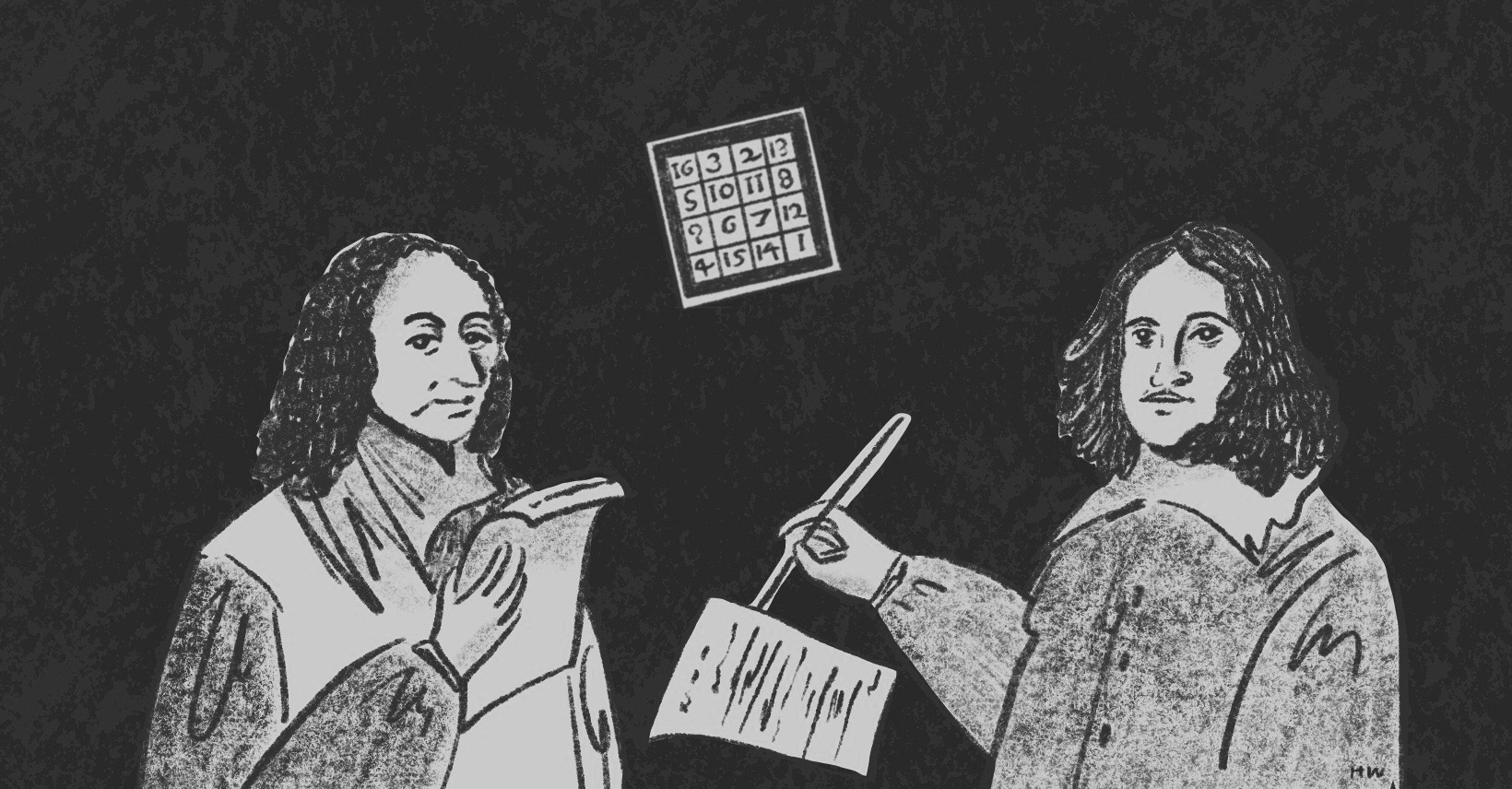×資料管理 統計分析 相關資源 巨人肩膀 語法索引 關於作者

## 簡介

• poly() -多項式回歸
• gam() -廣義相加模型
• plot.gam() -繪製GAM配適圖
• vis.gam() -繪製透視圖
• gam.check() -GAM模型診斷
• predict.gam() -GAM模型預測
• s() -平滑曲線
• loess() -局部回歸
• bs() -多項式樣條
• SMA() -簡單移動平均
• EMA() -指數移動平均
• legend() -圖例

## 為什麼不用線性回歸？

$$y=\alpha x + \beta$$是我們再熟悉不過的模型，但真實世界中更多是非線性相關。舉例來說，從中華民國統計資訊網的資料，我們可以畫出台灣1961至2020年超過半世紀的經濟成長率：

## 廣義相加模型

• 線性回歸模型：$$y=\beta_{0}+ \displaystyle\sum_{i=1}^n \beta_{i} x_{i} + \epsilon$$
• 廣義相加模型：$$y=\beta_{0}+ \displaystyle\sum_{i=1}^n s_{i}(x_{i}) + \epsilon$$

### 平滑曲線

• 移動平均(Running Mean)
• 移動平均是最簡單能將一條折線修勻的方法，最常應用在股票分析。一個簡單移動平均(Simple Moving Average)公式如下：$$F_{t+1}=\frac{過去n期的總數}{n}$$。也就是第$$t+1$$期的移動平均，等於過去n期的算術平均。指數平滑法是另一個常用的技巧，通常能比簡單移動平均的線條再平滑些，其公式為：$$F_{t+1}=D_{t} + \alpha(F_{t}-D_{t})$$。其概念是透過$$\alpha$$參數，來調控預測值$$F_{t}$$與實際值$$D_{i}$$的誤差。TTR套件中有SMA()、EMA()可以計算移動平均。手動計算則可進一步參考SMA & EMA Step by Step

• 局部回歸(Local Regression, Loess)
• 基本原理是透過局部的線性回歸，串聯起一條平滑曲線。其概念有點像移動平均，只不過這個移動平均現在被回歸所取代而已。例如在散布圖中三個相鄰的資料點$$(x_{1},y_{1})、(x_{2},y_{2})、(x_{3},y_{3})$$可以計算出一條回歸方程式，再根據這個回歸方程式計算出$$x_{2}$$所對應的$$y_{2}$$平滑值。然後依序再對$$(x_{2},y_{2})、(x_{3},y_{3})、(x_{4},y_{4})$$作回歸，計算$$y_{3}$$的平滑值，以此類推下去。

通常採用Loess必須先決定每一段回歸線的鄰近區域大小，才能知道要取那些點計算回歸方程式。在局部加權回歸中，也會決定出一個加權函數，給予比較近的資料點有較大的權重，遠處的資料點有較小的權值。詳細步驟可參考Loess Step by Step

• 樣條插值法(Spline Interpolation)
• 所謂插值法就是找一個函數，穿過所有給定的函數值。看起來就像是在相鄰的函數值之間，插滿函數值，因而這條函數也稱為內插函數。最熟悉的莫過於牛頓插值法(Newton polynomial interpolation)與拉格朗日插值法(Lagrange polynomial interpolation)，可參考插值法 Step by Step

插值可以使用分段的多項式進行插值，比較常用的有三次樣條插值(cubic spline interpolation)，其精神就在於兩個相鄰點之間，找出一個合理的三次多項式。概念如下圖並可以形成下列分段多項式。至於兩條相鄰曲線之間的銜接點─節點(knot)必須平滑，只要令其一階導函數和二階導函數值相等即可。

$S(x)= \begin{cases} s_{1}=\beta_{0} + \beta_{1} x^3 + \beta_{2} x^2 + \beta_{3} x\\ s_{2}=\beta_{0} + \beta_{1} x^3 + \beta_{2} x^2 + \beta_{3} x\\ s_{3}=\beta_{0} + \beta_{1} x^3 + \beta_{2} x^2 + \beta_{3} x \end{cases}$

在擬合三次樣條的過程中，當然不可能把所有的函數點都插值，我們希望擬合出來的線條能趨近真實資料的分布型態，不會出現過度擬合(overfitted)或擬合不足(underfitted)。為了避免這種情形，除了要考慮殘差的最小平方之外，還要考慮曲線的平滑程度。曲線波動太大(wiggling)，容易受到離群值得影響，降低模型解釋力；曲線太過平滑，又會失去曲線模型的意義。為了解決這個兩難，我們在最小平方的後面多加了一個「懲罰項」(penality)：$$\lambda\int(s''(x))^2\mathrm{d}x$$，所以模型擬合出來的曲線會變成：

$\sum_{i=1}^{n}(y_{i}-f(x_{i}))^2+\lambda\int(s''(x))^2\mathrm{d}x$

懲罰項是平滑曲線二階導數的積分。一階導數是曲線的斜率，二階導數是斜率的斜率，也就是斜率的變化率，換句話說如果曲線波動很大的話，二階導數也會很大，如果一條直線的話那麼二階導數為0。取積分的意義可以想像是把「斜率的變化率」面積加總，如此一來就可以衡量平滑曲線的波動程度。在數學涵義上，加入一個$$\lambda$$參數來控制懲罰項，讓我們更容易決定曲線的波動程度。當$$\lambda=0$$，懲罰項為0，擬合點過多造成曲線節點(knot)太多波動太大，也就是過度擬合；反之$$\lambda$$趨近於無限大，則會接近一條直線，也就是擬合不足。一般情況，我們會設定$$\lambda=0.6$$或是讓R自行調整。比較移動平均、局部回歸與三次樣條擬合的情形如下：

 > loess<-loess(growth~year, data=okun, span=0.6) #局部回歸模型 > fit_loess<-fitted(loess) #估計局部回歸模型的經濟成長率 > library(splines) #載入splines套件 > cubic<-lm(growth~bs(year, knots=6, degree=3), data=okun) #三次樣條模型 > fit_cubic<-fitted(cubic) #估計三次樣條模型的經濟成長率 > library(TTR) #載入TTR套件 > sma<-SMA(okun[,"growth"], n=10) #計算10年移動平均 > ema<-EMA(okun[,"growth"], ratio=0.3) #計算指數移動平均 > par(mfrow=c(2,2)) #設定圖表輸出版型為2x2 > plot(x=okun$year, y=okun$growth, type="l", main="Economic Growth", xlab="Year", ylab="Economic Growth %") #繪製折線圖 > lines(year, sma, col="red", lwd=2) #加上移動平均趨勢線 > legend("bottomleft", legend=c("SMA"), col=c("red"), lty=1, lwd=2) #圖例 > plot(x=okun$year, y=okun$growth, type="l", main="Economic Growth", xlab="Year", ylab="Economic Growth %") #繪製折線圖 > lines(year, ema, col="blue", lwd=2) #加上指數移動平均趨勢線 > legend("bottomleft", legend=c("EMA"), col=c("blue"), lty=1, lwd=2) #圖例 > plot(x=okun$year, y=okun$growth, type="l", main="Economic Growth", xlab="Year", ylab="Economic Growth %") #繪製折線圖 > lines(year, fit_loess, col="purple", lwd=2) #加上局部回歸趨勢線 > legend("bottomleft", legend=c("Loess"), col=c("purple"), lty=1, lwd=2) #圖例 > plot(x=okun$year, y=okun$growth, type="l", main="Economic Growth", xlab="Year", ylab="Economic Growth %") #繪製折線圖 > lines(year, fit_cubic, col="darkgreen", lwd=2) #加上三次樣條趨勢線 > legend("bottomleft", legend=c("Cubic Spline"), col=c("darkgreen"), lty=1, lwd=2) #圖例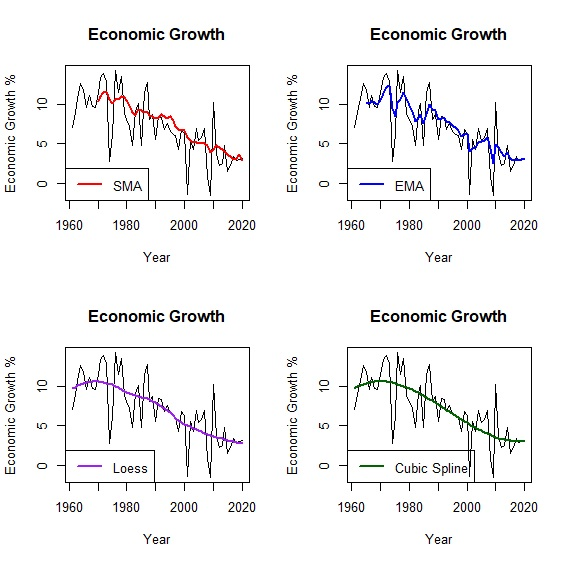### 模型試作

 > plot(x=unemployment, y=growth, main="Taiwan's Okun Law", xlab="Unemployment %", ylab="Economic Growth %")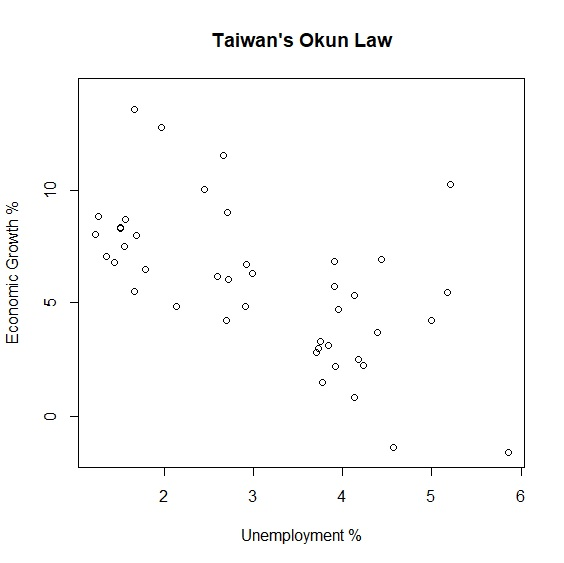> library(mgcv) #載入mgcv套件 > okun_new<-okun[complete.cases(okun),] > attach(okun_new) > gam<-gam(growth~s(unemployment), data=okun_new) #廣義相加模型 > summary(gam) Family: gaussian Link function: identity Formula: growth ~ s(unemployment) Parametric coefficients: Estimate Std. Error t value Pr(>|t|) (Intercept) 5.8447 0.3786 15.44 <2e-16 *** --- Signif. codes: 0 ‘***’ 0.001 ‘**’ 0.01 ‘*’ 0.05 ‘.’ 0.1 ‘ ’ 1 Approximate significance of smooth terms: edf Ref.df F p-value s(unemployment) 5.202 6.296 5.773 0.000209 *** --- Signif. codes: 0 ‘***’ 0.001 ‘**’ 0.01 ‘*’ 0.05 ‘.’ 0.1 ‘ ’ 1 R-sq.(adj) = 0.45 Deviance explained = 51.8% GCV = 7.2019 Scale est. = 6.1632 n = 43 

GAM的輸出報表大致分為五個部分。

2. 模型的連結函數。現在這個模型是高斯分配(gaussian)，但如果應變數是類別變數，GAM其實也可以改為其他類型的分配。

3. 方程式 (Formula)
4. 說明模型之間的變數關係，也就是方程式長什麼樣子。

5. 參數 (parametric coefficients)
6. 這是我們熟悉的部分，和過去回歸模型的內容一樣，可以看到常數項是5.8447。

7. 平滑曲線 (smooth terms)
8. GAM最重要的部分。可以看到smooth term已經沒有預測參數，而是以effective degrees of freedom(edf)來代替。edf是GAM的統計量，反應的是非線性的程度，通常edf越大代表模型越複雜，非線性的情況越明顯；反之當edf=1的時候，代表兩者是線性相關，也就是跟一般的回歸模型一樣。

9. 解釋力
10. 最後一部分是模型解釋力，可以看到$$R^2=0.45$$。

plot.gam()輸出廣義相加模型的平滑曲線如下：

 > plot.gam(gam, residuals=TRUE, pch=1, shade=TRUE, shade.col="lightblue")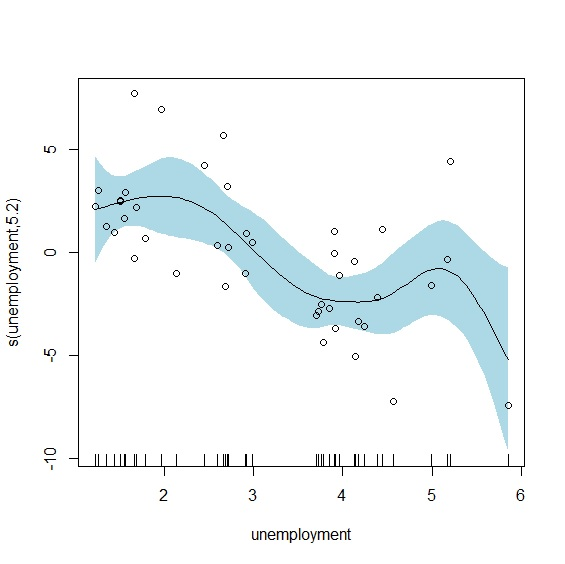gam.check()輸出模型檢定報表。報表第一段說明檢定的方法是「廣義交叉驗證」(Generalised Cross-Validation, GCV)，GCV是用來選擇平滑參數$$\lambda$$的指標，與AIC一樣GCV的數值越小表示模型越佳。gam()會自動透過最小誤差或最大概似法，替每個模型找到適合的平滑參數。GCV的原理是誤差極小化來決定平滑參數，這也是gam()預設的方法。但多數人認為GCV模型有平滑不足(undersmoothing)的問題，因此更推薦使用有限最大概似法(restricted maximum likelihood, REML)來決定平滑參數。

 > gam.check(gam) Method: GCV Optimizer: magic Smoothing parameter selection converged after 5 iterations. The RMS GCV score gradient at convergence was 0.0003435376 . The Hessian was positive definite. Model rank = 10 / 10 Basis dimension (k) checking results. Low p-value (k-index<1) may indicate that k is too low, especially if edf is close to k'. k' edf k-index p-value s(unemployment) 9.0 5.2 1.42 0.99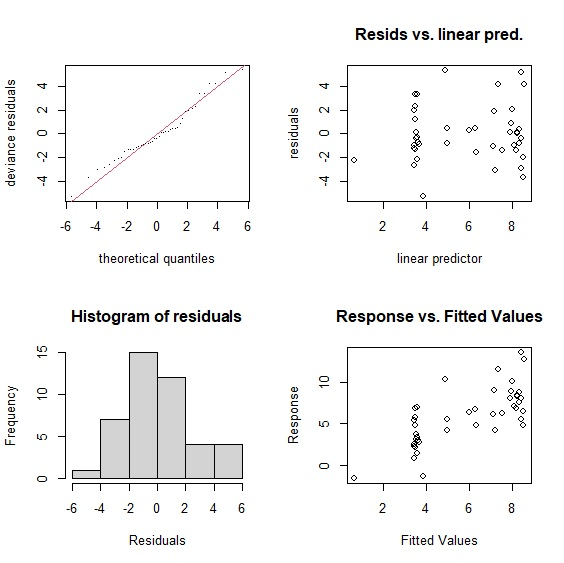> linear<-lm(growth~unemployment, data=okun) > poly<-lm(growth~poly(unemployment, degree=2, raw=TRUE), data=okun) > cubic<-lm(growth~bs(unemployment), data=okun) > summary(linear)$r.squared  0.37149 > summary(poly)$r.squared  0.3743529 > summary(cubic)$r.squared  0.3837308 > summary(gam)$r.sq  0.450145 > AIC(linear)  210.9652 > AIC(poly)  212.7689 > AIC(cubic)  214.1195 > AIC(gam)  207.9345 
Model $$R^2$$ AIC
Linear 0.37 210.97
Polynomial 0.37 212.77
Cubic 0.38 214.12
GAM 0.45 207.93

 > anova(linear, gam, test="Chisq") Analysis of Variance Table Model 1: growth ~ unemployment Model 2: growth ~ s(unemployment) Res.Df RSS Df Sum of Sq Pr(>Chi) 1 41.000 295.88 2 36.798 226.79 4.202 69.089 0.02812 * > anova(poly, gam, test="Chisq") Analysis of Variance Table Model 1: growth ~ poly(unemployment, degree = 2, raw = TRUE) Model 2: growth ~ s(unemployment) Res.Df RSS Df Sum of Sq Pr(>Chi) 1 40.000 294.53 2 36.798 226.79 3.202 67.741 0.01411 * > anova(cubic, gam, test="Chisq") Analysis of Variance Table Model 1: growth ~ bs(unemployment) Model 2: growth ~ s(unemployment) Res.Df RSS Df Sum of Sq Pr(>Chi) 1 39.000 290.12 2 36.798 226.79 2.202 63.326 0.00741 ** 

 > linear<-lm(growth~unemployment, data=okun_new) > poly<-lm(growth~poly(unemployment, degree=2, raw=TRUE), data=okun_new) > cubic<-lm(growth~bs(unemployment), data=okun_new) > fit_poly<-fitted(poly) > fit_cubic<-fitted(cubic) > par(mfrow=c(2,2)) > plot(x=unemployment, y=growth, main="Linear", xlab="Unemployment %", ylab="Economic Growth %") > abline(lm(growth~unemployment)) > plot(x=unemployment, y=growth, main="Polynomial", xlab="Unemployment %", ylab="Economic Growth %") > lines(unemployment, fit_poly) > plot(x=unemployment, y=growth, main="Cubic Spline", xlab="Unemployment %", ylab="Economic Growth %") > lines(unemployment, fit_cubic) > plot.gam(gam, residuals=TRUE, main="GAM")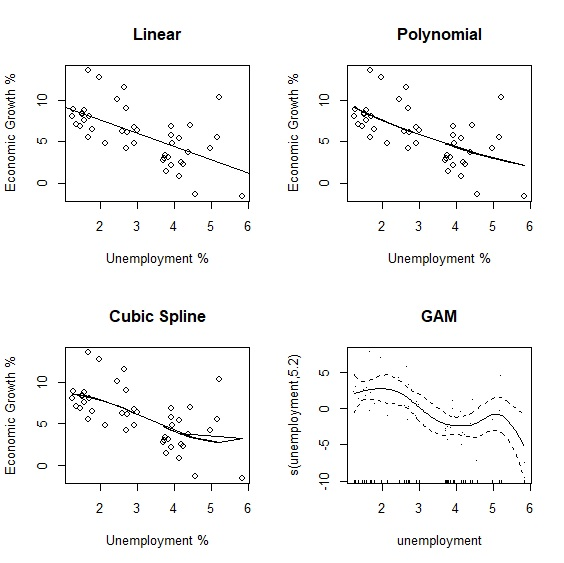> var.unemployment<-data.frame(unemployment=c(4.80,3.91,3.73,3.71,3.76)) > predict.gam(gam, newdata=var.unemployment) 1 2 3 4 5 4.561867 3.490964 3.641897 3.669994 3.604767 

### 交互作用

 > gam2<-gam(growth~s(save)+s(investment)+s(save, investment), data=okun, method="REML") > summary(gam2) Family: gaussian Link function: identity Formula: growth ~ s(save) + s(investment) + s(save, investment) Parametric coefficients: Estimate Std. Error t value Pr(>|t|) (Intercept) 7.1472 0.3177 22.5 <2e-16 *** --- Signif. codes: 0 ‘***’ 0.001 ‘**’ 0.01 ‘*’ 0.05 ‘.’ 0.1 ‘ ’ 1 Approximate significance of smooth terms: edf Ref.df F p-value s(save) 5.214 6.183 2.866 0.0158 * s(investment) 3.188 3.844 2.897 0.0368 * s(save,investment) 2.107 27.000 0.112 0.0729 . --- Signif. codes: 0 ‘***’ 0.001 ‘**’ 0.01 ‘*’ 0.05 ‘.’ 0.1 ‘ ’ 1 R-sq.(adj) = 0.591 Deviance explained = 66.4% -REML = 147.97 Scale est. = 6.0548 n = 60 

plot()輸出模型擬合結果，因為有三個自變數，因此共會輸出三張圖表。左上是儲蓄率的平滑曲線，右上是投資率的平滑曲線，下圖則是以等高線圖(contour)來呈現兩者的交互作用。從圖形來看，儲蓄率對經濟成長率的影響是先下降再上升，而投資率則是先上升後下降。至於交互作用項從等高線圖難以判別，我們可以改用3D圖來觀察。

 > layout(matrix(c(1,2,3,3), 2, 2, byrow=TRUE)) > plot(gam2)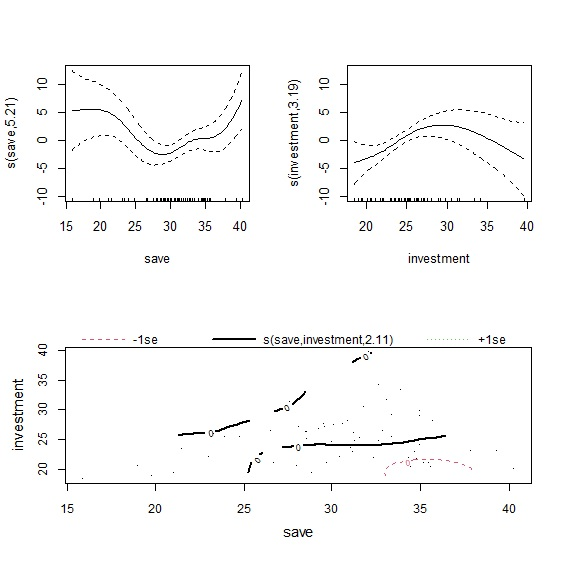> plot(gam2, scheme=1)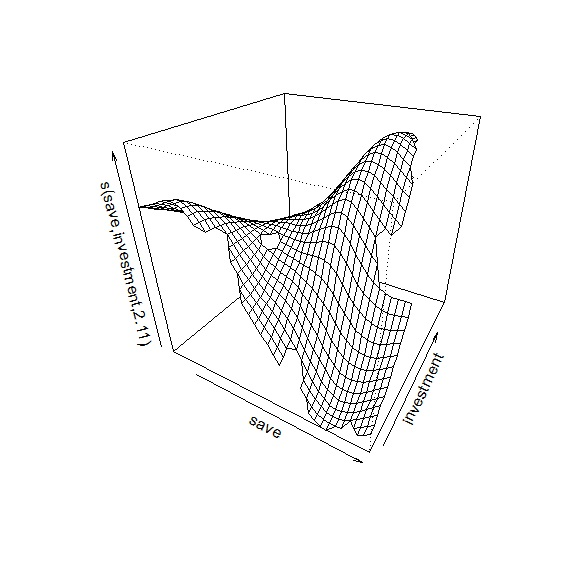> vis.gam(gam2, view=c("save", "investment"), plot.type="persp")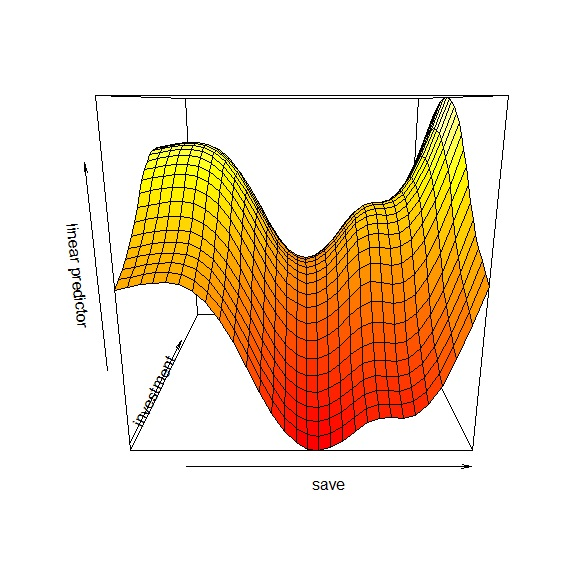$$s(x_{1}, x_{2})$$當作交互作用項的時候，整個平滑曲線只有一個$$\lambda$$參數，當$$x_{1}$$與$$x_{2}$$單位相同的時候，共用$$\lambda$$參數並無不可，不過如果兩個變數單位不同的時候，共用同一個$$\lambda$$參數就顯得有些奇怪了。這時候就可以引進tensor來建構模型。用下面的方程式更能瞭解兩種交互作用模型的差異：

$\text{原始模型： }y=s(x_{1})+s(x_{2})+s(x_{1}, x_{2}) \text{ with } \lambda_{1}, \lambda_{2}, \lambda_{3}$

$\text{Tensor模型： }y=s(x_{1})+s(x_{2})+ti(x_{1}, x_{2}) \text{ with } \lambda_{1}, \lambda_{2}, \lambda_{3}, \lambda_{4}$